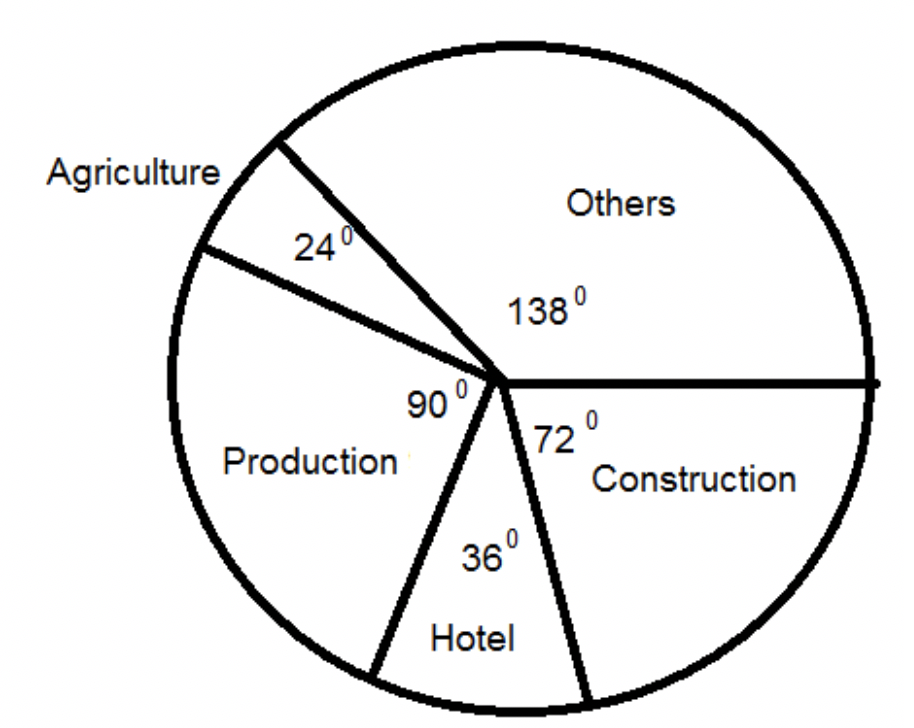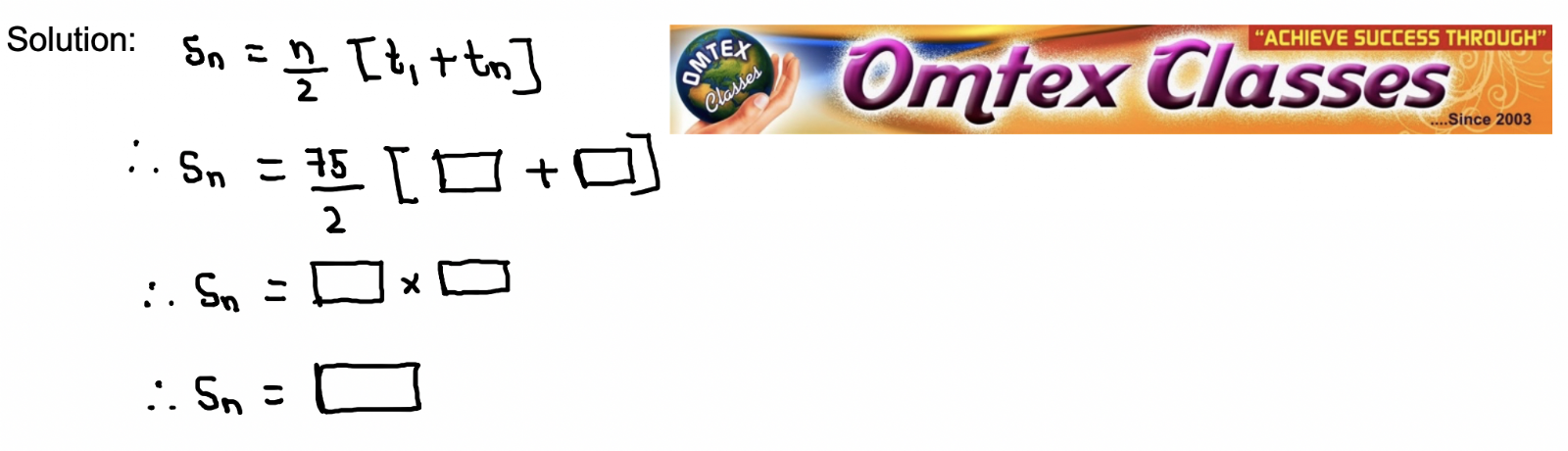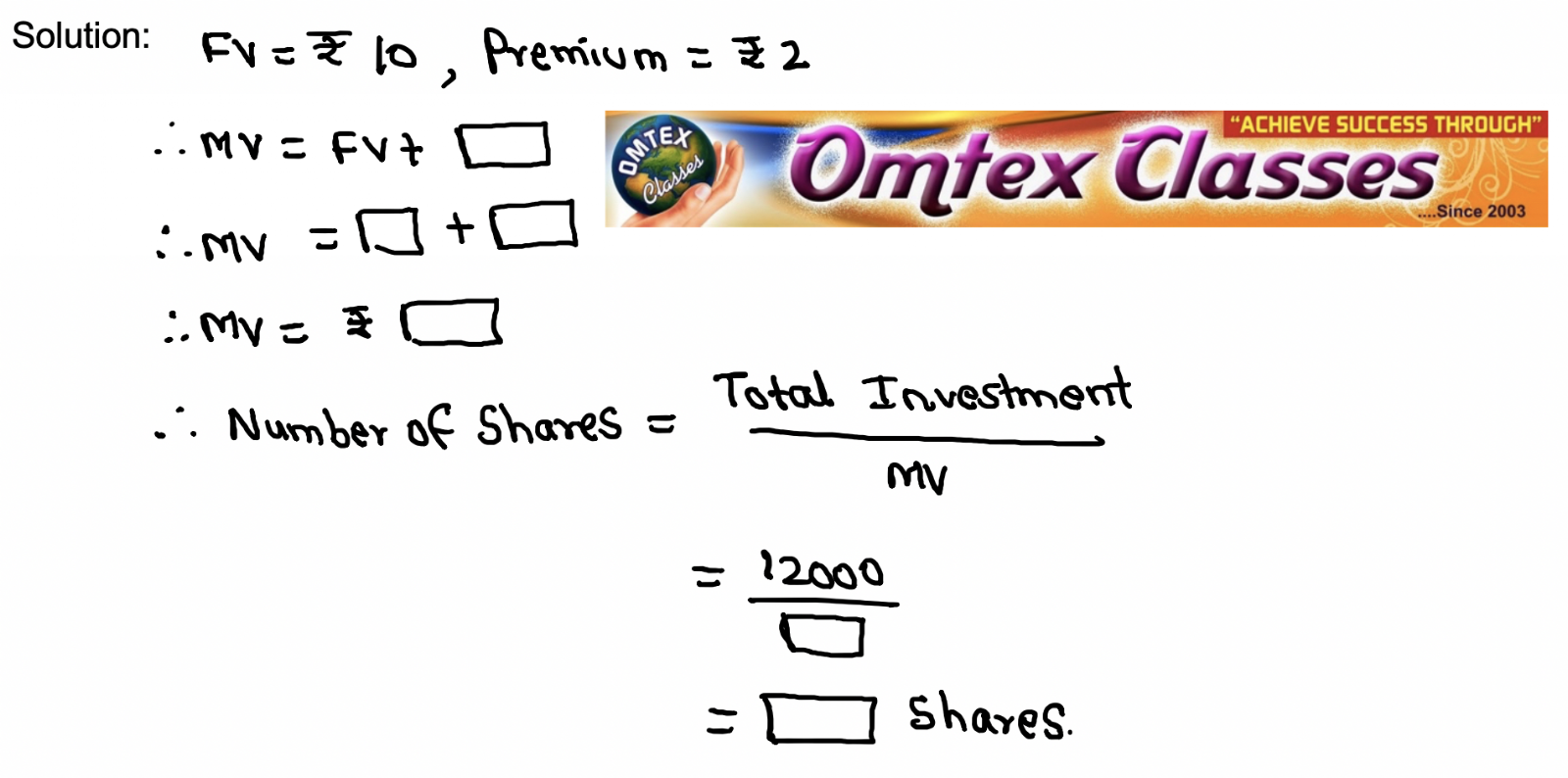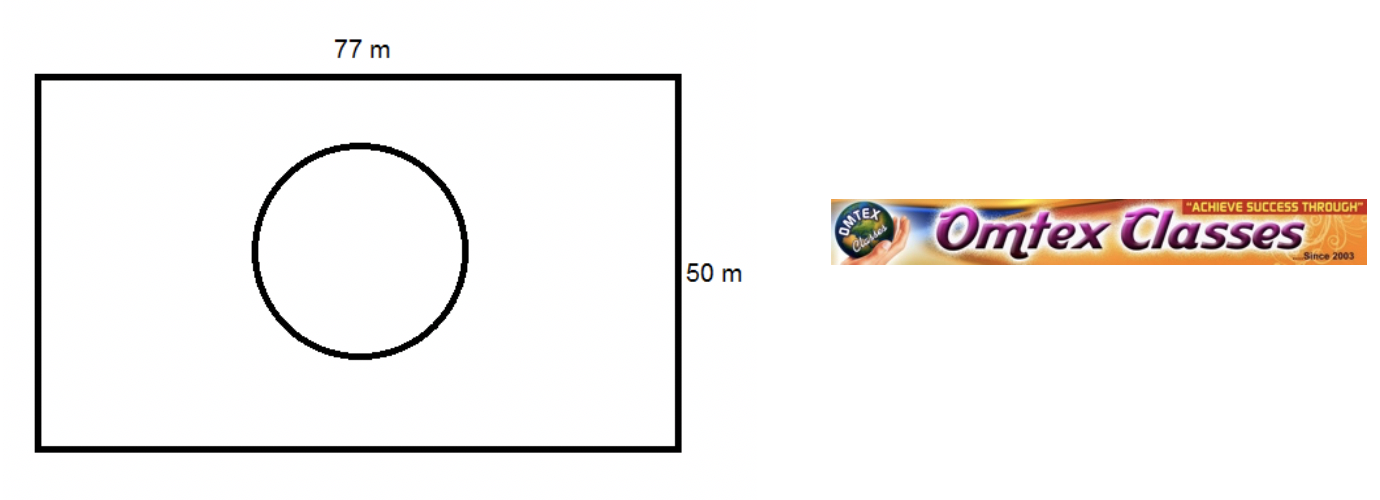### Important Maths 1 Question Paper For Board Exam 2019 SSC New Syllabus.

Mathematics – I      Paper No. 2
Time : 2Hrs.               Marks: 40
Q. 1. A. Solve the following questions. (Any 4)             [4 Marks]
1. If M = {1, 3, 5} , N = {2, 4, 6} then M ⋂ N = ?
2. State the order of the surds √39.
3. Write the coefficient of m3 in the following polynomial: - 3 + m - √3 m3

4. Find the ratio of the first number to the second number, in the reduced form: 72, 60.
5. By using variables x and y form any five linear equations in two variables.
6. How much secondary and higher secondary cess at 1% is levied on income tax Rs. 50,000?
B. Solve the following questions. (Any 2) 
1. Find the mean of the following numbers: 87, 92, 63, 78, 92, 59, 72, 66, 69
2. Find the ratio of the first quantity to the second quantity in the reduced form: 2 min 6 sec, 3 min 54 sec.
3. Add the following polynomials: 3x2 + 5x – 4; 8x – 2x2 + 11
Q. 2. A. Choose the correct alternative: [4 marks]
1. One of the roots of equation x2 + mx – 5 = 0 is 2; find m.
A) – 2 B) – ½       C) ½    D) 2
2. 15, 10, 5, … in this A.P. sum of first 10 terms is _______
A) – 75 B) – 125 C) 75 D) 125
3. In the format of GSTIN there are ______ alpha – numerals.
A)15 B) 10   C) 16 D) 9
4. The probability getting a prime number in single throw of a dice is _________
A) zero B) 1/3 C) 1/2   D) 1/4
B. Solve the following questions. (Any 2) [4 Marks]
1) If one coin and one die are thrown simultaneously then find the probability of getting head and prime number.
2) As deduced from a survey, the classification of skilled workers is shown in the pie diagram. If the number of workers in the production sector is 4500, then what is the total number of skilled workers in all fields.3) The age group and number of persons, who donated blood in a blood donation camp is given below. Draw a pie diagram from it.
 Age Group (years) 20 – 25 25 – 30 30 – 35 35 – 40 No. of Persons 80 60 35 25
Q. 3. (A) Complete the following activities. (Any 2) [4 Marks]
1) Find the sum of these 75 numbers. 1 + 3 + 5 + ….. + 149.2) Smita has invested Rs. 12,000 and purchased shares of FV Rs. 10 at a premium of Rs. 2. Find the number of shares she purchased. Complete the given activity to get the answer.3) Complete the following activity.

There are 52 cards in a pack.
In each pack, total black face cards are ________________
There are ______________ kings of red colour.
There are ______________ cards which are not of diamond.
In each pack, total club cards are __________________.
B) Solve the following questions (Any 2)
1) Solve the following quadratic equation: 5x2 – 4x – 7 = 0.

2) How many two digit numbers are divisible by 4?

3) The weekly wages of 120 workers in a factory are shown in the following frequency distribution table. Find the mean of the weekly wages.
 Weekly Wages (Rs.) 0 – 2000 2000 – 4000 4000 – 6000 6000 – 8000 No. of Workers 15 35 50 20
Q. 4. Solve the following questions (Ans 3)

1. A certain amount is equally distributed among certain number of students. Each would get Rs. 2 less if 10 students were more and each would get Rs. 6 more. If 15 students were less. Find the number of students and the amount distributed.

2. There are 37 terms in an A.P., the sum of three terms placed exactly at the middle is 225, and the sum of last three terms is 429. Write the A.P.

3. Shruti invested Rs. 6024 in the share of FV of Rs. 10 when the market value was Rs. 60. She sold all the shares at MV of Rs. 50 after taking 60% dividend. She paid 0.4% brokerage at each stage of transactions. What was the total gain or loss in this transaction?

4. Length and breadth of a rectangular garden are 77 m and 50 m respectively. There is a circular lake in the garden having diameter 14 m. Due to wind, a towel from a terrace on a nearby building fell into the garden. Then find the probability of the event that it fell in the lake.Q. 5. Solve the following questions. (Any 1)

1. Two tractors, one moving towards north and the other towards east, leave the same place at the same time. The speed of one of them is greater than that of the other by 5 km/hr. At the end of two hours they are at a distance of 50 km from each other. Find the speed of each tractor.

2. Draw the graphs representing the equations 2x = y + 2 and 4x + 3y = 24 on the same graph paper. Find the are of the triangle formed by these lines and the X – axis.

Q. 6. Solve the following questions. (Any 1) (3 Marks)

1. The sum of father’s age and twice the age of his son is 70. If we double the age of the father and add it to the age of his son, the sum is 95. Find their present ages.

2) A two-digit number is to be formed from the digits 0, 1, 2, 3, 4. Repetition of the digits is allowed. Find the probability that the number so formed is a ________

(a) multiple of 4        (b) prime number.